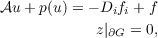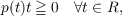#### Vol. 57, No. 1, 1975

 Download this articleFor screen For printingRecent Issues Vol. 307: 1  2 Vol. 306: 1  2 Vol. 305: 1  2 Vol. 304: 1  2 Vol. 303: 1  2 Vol. 302: 1  2 Vol. 301: 1  2 Vol. 300: 1  2Online Archive Volume: Issue:The Journal Officers Subscriptions Editorial Board Submission Guidelines Submission Form Contacts ISSN: 1945-5844 (e-only) ISSN: 0030-8730 (print) Special Issues Author Index To Appear Other MSP Journals
On bounded solutions of a strongly nonlinear elliptic equation

### Nguyên Phuong Các

Vol. 57 (1975), No. 1, 53–58
##### Abstract

I. Introduction. Consider the Dirichlet problem for a bounded domain G Rn(n 2) having smooth boundary ∂G:(1)

where 𝒜 is a second order differential operator of Leray-Lions type mapping a real Sobolev space W01q(G)(1 < q < ) into its dual; f,fi(i = 1,,n) are given functions. We have used the notation Di for the derivative in the distribution sense ∂∕∂xi and the convention that if an index is repeated then summation over that index from 1 to n is implied. We shall assume that the real function p(t) is continuous and satisfies the condition(2)

but otherwise (p)t is not subject to any growth condition.

In this paper we discuss the existence of a solution of equation (1) in W01,q(G) L(G).

Primary: 35J65
##### Milestones